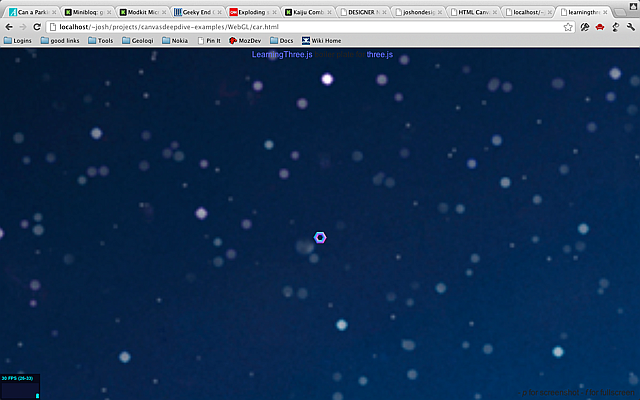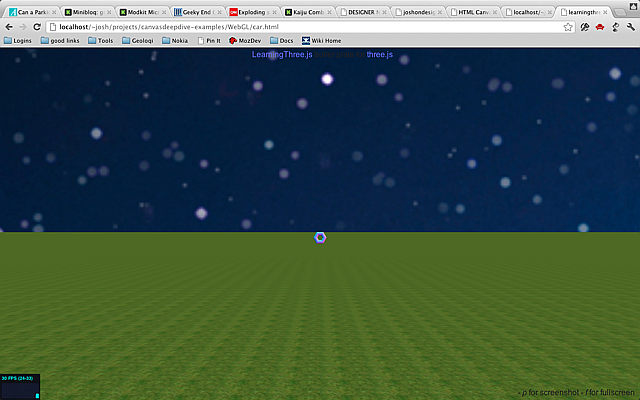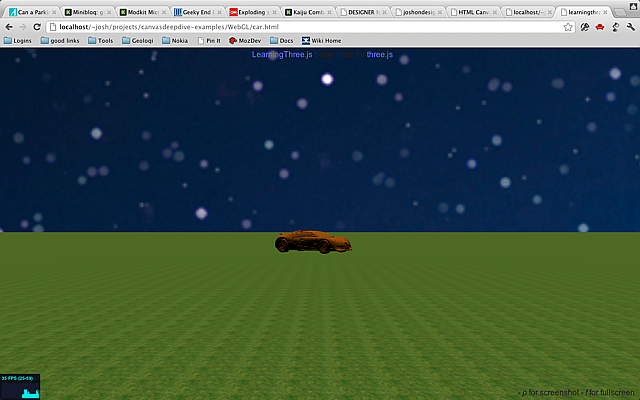### Building A Sky

For our hands on, we will create a new scene: a car that drives around on a large grassy plain under a starry sky. This is adapted from a series of great blog posts by Jerome, who also created the template builder and tQuery, which is like JQuery, but for ThreeJS. (original series.)

Start with a new template from the template builder. Now let's add a sky. The easy way to make a sky is to just put sky pictures on the sides of a big cube. The trick is that we will put the rest of the world inside of the cube. We will start by loading up images into a single cube texture like this:

``````//add skymap
var urls = [
"images/sky1.png",
"images/sky1.png",
"images/sky1.png",
"images/sky1.png",
"images/sky1.png",
"images/sky1.png",
];

The image `sky1.png` is included in the example code download.

Now we need a cube shader to draw it with standard uniforms (shader inputs.) Notice that we've set the `tCube` texture to be our texture.

``````//setup the cube shader
uniforms['tCube'].texture = textureCube;
uniforms       : uniforms
});
``````

Now, we need a cube geometry. Set the size to `10000`. This will be a big cube. Now we add it to the scene. We set `flipSided` to true because a default cube has the texture drawn on the outside. In our case we are on the inside of the cube.

``````//create a skybox
var size = 10000;
skyboxMesh = new THREE.Mesh(
new THREE.CubeGeometry(size,size,size),material);
//IMPORTANT!! draw on the inside instead of outside
skyboxMesh.flipSided = true; // you must have this or you won't see anything
``````

Now let's add a light from the sun. Without a light we cannot not see anything.

``````//add sunlight
var light = new THREE.SpotLight();
light.position.set(0,500,0);

Here's what we've got so far:Now we want a ground plane. First you need to load the grass image (original source) as a texture. (The grass image is also included in the example code.) Set it to repeat in the x and y directions. The repeat values should be the same as the size of the texture, and usually should be a power of two (ex: 256).

``````//add ground
grassTex.wrapS = THREE.RepeatWrapping;
grassTex.wrapT = THREE.RepeatWrapping;
grassTex.repeat.x = 256;
grassTex.repeat.y = 256;
var groundMat = new THREE.MeshBasicMaterial({map:grassTex});``````

Next is the geometry. It is just a big plane in space. The size of the plane is 400 x 400 which is fairly large compared to the camera but very small relative to the size of the sky, which is set to 10000.

``var groundGeo = new THREE.PlaneGeometry(400,400);``

Now we can combine them into a mesh. Set `position.y` to -1.9 so it will be below the torus. Set `rotation.x` to 90 degrees so the ground will be horizontal (a plane is vertical by default.) If you can't see, the plane try setting `doubleSided` to true. Planes only draw on a single side by default.

``````var ground = new THREE.Mesh(groundGeo,groundMat);
ground.position.y = -1.9; //lower it
ground.rotation.x = -Math.PI/2; //-90 degrees around the xaxis
//IMPORTANT, draw on both sides
ground.doubleSided = true;

Here's what it should look like now:To replace the torus with a car we will load an external model, in this case a very detailed model of a Bugatti Veyron created by Troyano . I got these from the ThreeJS examples repo. You can find them in the example code download. Since this model is in a binary format rather than JSON, we will load it up using the `THREE.BinaryLoader`.

``````//load a car
//IMPORTANT: be sure to use ./ or it may not load the .bin correctly
var orange    = new THREE.MeshLambertMaterial( { color: 0x995500, opacity: 1.0, transparent: false } );
var mesh	= new THREE.Mesh( geometry, orange );
mesh.scale.x = mesh.scale.y = mesh.scale.z = 0.05;
car = mesh;
});``````

Notice that the material is a `MeshLambertMaterial` rather than the `MeshNormalMaterial` we used before. This will give the car a nice solid color that is properly shaded based on the light (orange, in this case). This mesh is huge by default compared to the torus, so scale it down to 5%, then add it to the scene.

Here's what it looks like now:### Keyboard Control

Of course a car just sitting there is no fun. And it's too far away. Let's make it move. Currently the `cameraControl` object is moving the camera around. Remove that and create a new `KeyboardState` object where the `cameraControl` object was initialized. You will need to import `vendor/threex/THREEx.KeyboardState.js` at the top of your page.

``<script src="vendor/threex/THREEx.KeyboardState.js"></script>``
``````// create a camera contol
//cameraControls	= new THREEx.DragPanControls(camera)
keyboard = new THREEx.KeyboardState();``````

Now, go down to the `render()` function. The keyboard object will let us query the current state of the keyboard. To move the car around using the keyboard replace `cameraControls.update()` with this code:

``````// update camera controls
//cameraControls.update();
if(keyboard.pressed("left")) {
car.rotation.y += 0.1;
}
if(keyboard.pressed("right")) {
car.rotation.y -= 0.1;
}
if(keyboard.pressed("up")) {
car.position.z -= 1.0;
}
if(keyboard.pressed("down")) {
car.position.z += 1.0;
}``````

Now the car is "driveable" using the keyboard. Of course it doesn't look very realistic. The car can slide sideways. To fix it we need a vector to represent the current direction of the car. Add an `angle` variable and change the code to look like this:

``````if(keyboard.pressed("left")) {
car.rotation.y += 0.1;
angle += 0.1;
}
if(keyboard.pressed("right")) {
car.rotation.y -= 0.1;
angle -= 0.1;
}
if(keyboard.pressed("up")) {
car.position.z -= Math.sin(-angle);
car.position.x -= Math.cos(-angle);
}
if(keyboard.pressed("down")) {
car.position.z += Math.sin(-angle);
car.position.x += Math.cos(-angle);
}``````

### Next Steps

That's it for this hands on. If you wish to continue working with this example, here are a few things you might want to add.

• Make the camera follow the car.
• Make the car shiny. Look at the source to the original example that this was based on. [link].
• Make the car stop when you reach the edge of the world.
• Add the dot screen effect from the previous chapter to this scene.

You can view the final version here.

ThreeJS documentation